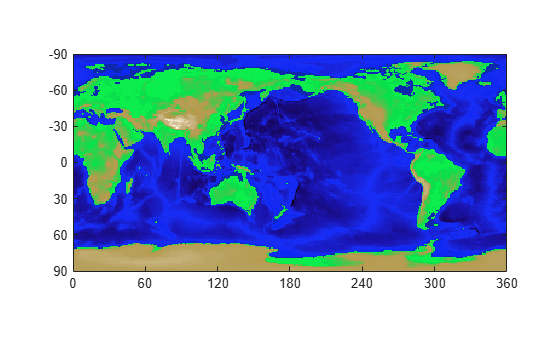# Displaying Topographic Data

This example shows several ways to represent the Earth's topography. The data used in this example are available from the National Geophysical Data Center, NOAA US Department of Commerce under data announcement 88-MGG-02.

The data file, `topo.mat`, contains topographic data. `topo` is the altitude data and `topomap1` is a colormap for the altitude.

```load topo topo topomap1 % load data whos('topo','topomap1')```
``` Name Size Bytes Class Attributes topo 180x360 518400 double topomap1 64x3 1536 double ```

### Create Contour Plot

One way to visualize topographic data is to create a contour plot. To show the outline of the Earth's continents, plot points that have zero altitude. The first three input arguments to `contour` specify the X, Y, and Z values on the contour plot. The fourth argument specifies the contour levels to plot.

```x = 0:359; % longitude y = -89:90; % latitude figure contour(x,y,topo,[0 0]) axis equal % set axis units to be the same size box on % display bounding box ax = gca; % get current axis ax.XLim = [0 360]; % set x limits ax.YLim = [-90 90]; % set y limits ax.XTick = [0 60 120 180 240 300 360]; % define x ticks ax.YTick = [-90 -60 -30 0 30 60 90]; % define y ticks```### View Data as Image

You can create an image of the topography using the elevation data and a custom colormap. The topography data is treated as an index into the custom colormap. Set the `CDataMapping` of the image to `'scaled'` to linearly scale the data values to the range of the colormap. In this colormap, the shades of green show the altitude data, and the shades of blue represent depth below sea level.

```image([0 360],[-90 90], flip(topo), 'CDataMapping', 'scaled') colormap(topomap1) axis equal % set axis units to be the same size ax = gca; % get current axis ax.XLim = [0 360]; % set x limits ax.YLim = [-90 90]; % set y limits ax.XTick = [0 60 120 180 240 300 360]; % define x ticks ax.YTick = [-90 -60 -30 0 30 60 90]; % define y ticks```### Use Texture Mapping

Texture mapping maps a 2-D image onto a 3-D surface. To map the topography to a spherical surface, set the color of the surface, specified by the `CData` property, to the topographic data and set the `FaceColor` property to `'texturemap'`.

```clf [x,y,z] = sphere(50); % create a sphere s = surface(x,y,z); % plot spherical surface s.FaceColor = 'texturemap'; % use texture mapping s.CData = topo; % set color data to topographic data s.EdgeColor = 'none'; % remove edges s.FaceLighting = 'gouraud'; % preferred lighting for curved surfaces s.SpecularStrength = 0.4; % change the strength of the reflected light light('Position',[-1 0 1]) % add a light axis square off % set axis to square and remove axis view([-30,30]) % set the viewing angle```